# Variables

## Variables

Variables are simply names that we can give to values such as strings, numbers and booleans. We use variables to store information that the computer can keep track of.

Here’s how to declare a variable named `str`. We say `str` has the value `"Hello World"`. Can you describe what the other variables below mean?

``````var str = "Hello World";
var x = 88;
var happy = true;
``````

Press run. Note that variables are not printed out to the console. Instead, the variable simply stores the string or number or boolean into the computer’s memory.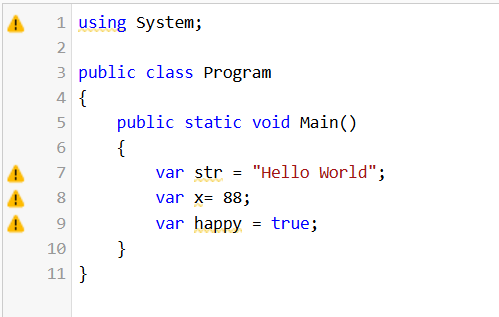We can use these variables in other statements. For example, the following code would print `Hello Nuevo Foundation` to the console:

``````var str1 = "Hello";
var str2 = "Nuevo Foundation";
Console.WriteLine(str1 + " " + str2);
``````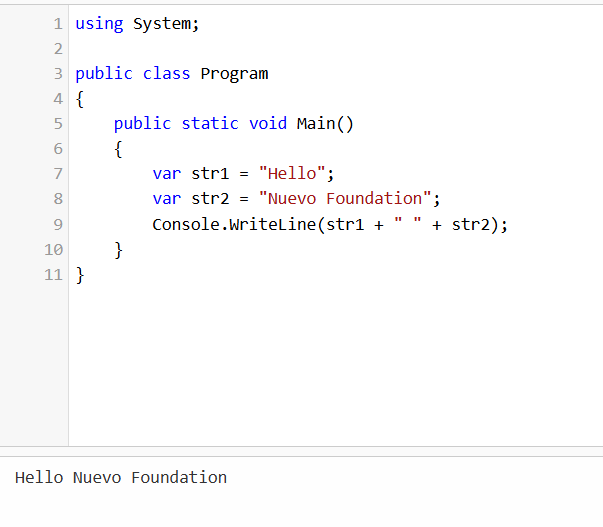You can change the value of a variable at any time by using the `=` operator again. If you’re changing a value of a variable, you don’t need to use `var` again. In the following example, `Hola` will be printed instead of `Hello`.

``````var str = "Hello";
str = "Hola";
Console.WriteLine(str);
``````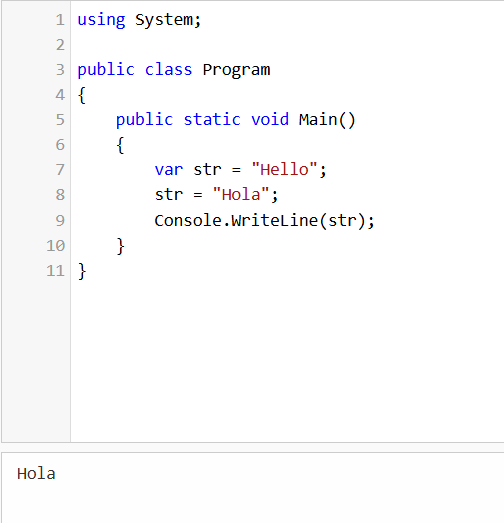Here’s another example: `10` will be printed instead of `9`.

``````var num = 9;
num = num + 1;
Console.WriteLine(num);
``````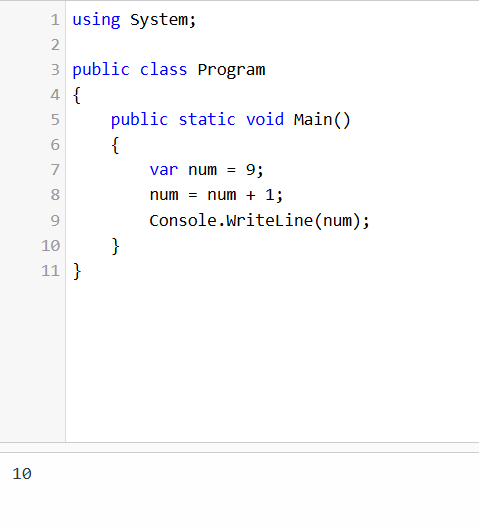Note that you CANNOT replace a string variable with a number or a boolean, and similarly for other combinations. The following example code will NOT work:

``````var happy = "Hello";
happy = 9;
``````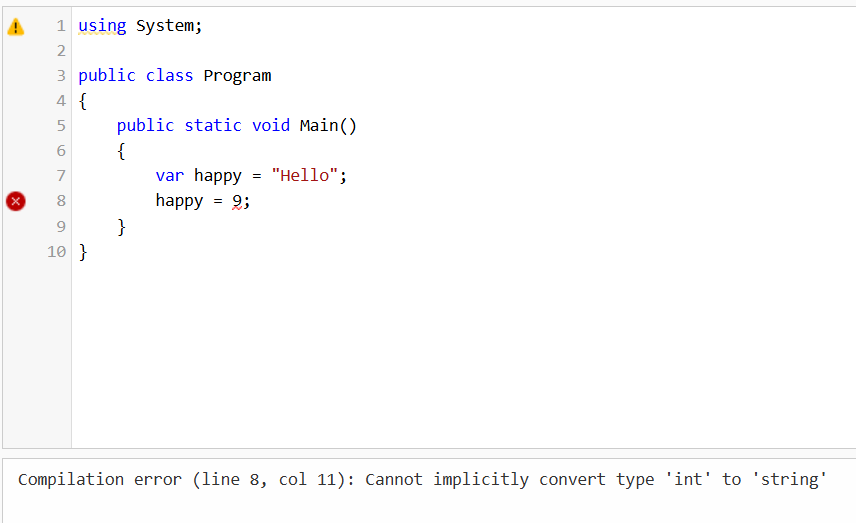Another way to declare variables is to replace var with the type (`string`, `int`, `bool`) of variable you are creating. By declaring the type explicitly, you avoid the problem that we see above. For example:

``````string str = "Hello World";
int x = 88;
bool happy = true;
``````

Note that `var`, `string`, `int`, or `bool` are special keywords in C#, so you cannot create variables with those names.